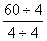﻿ What is a unit rate | Definition and Examples of unit rare | define unit rate in math | unit rate math worksheets – Algebra

## Definition Of Unit Rate

Unit Rate is the ratio of two measurements in which the second term is 1.

### More About Unit of Measurement

The two measurements involved for which ratio are taken in unit rate is always different.

### Example of Unit Rate

If Nancy earns $180 in 20 hours, then unit rate of her earning is given as 180/20 =$9 per hour.

### Solved Example on Unit Rate

#### Ques: William can pack 60 toys in 4 hours. Find the unit rate with which he packs toys.

##### Choices:

A. 16 toys/hour
B. 15 toys/hour
C. 14 toys/hour
D. 13 toys/hour

Step 3: =[Divide both the numerator and the denominator by the denominator.]